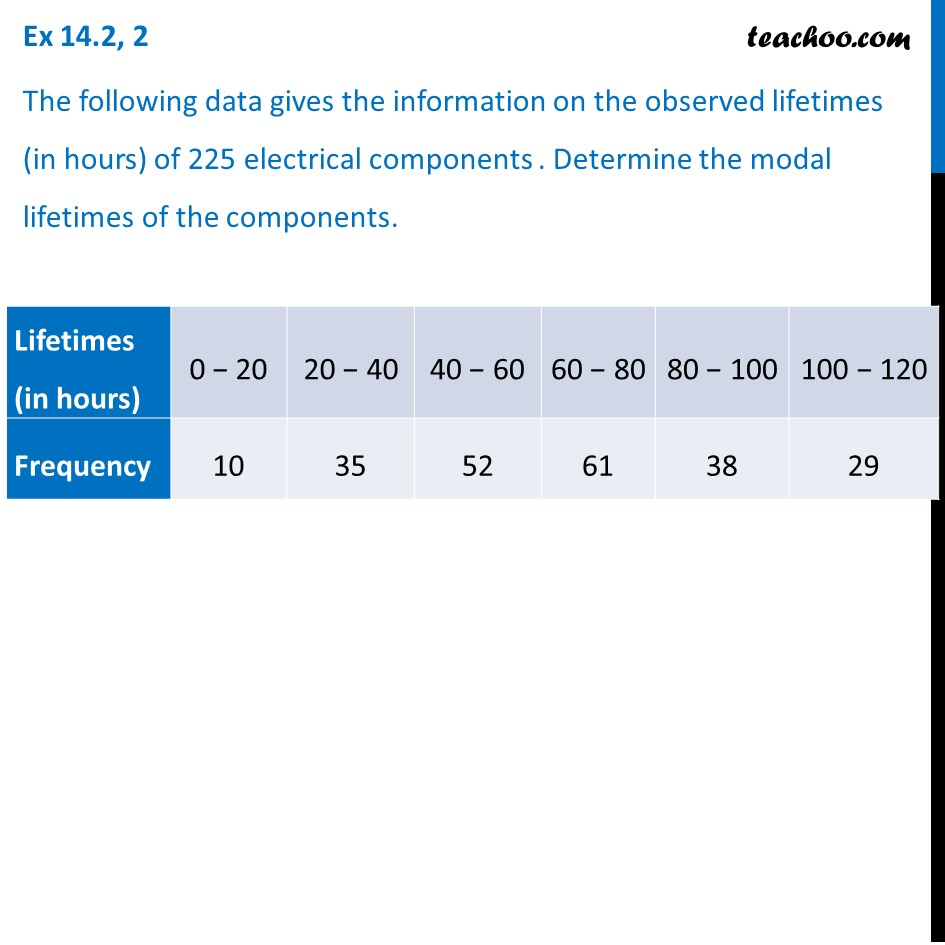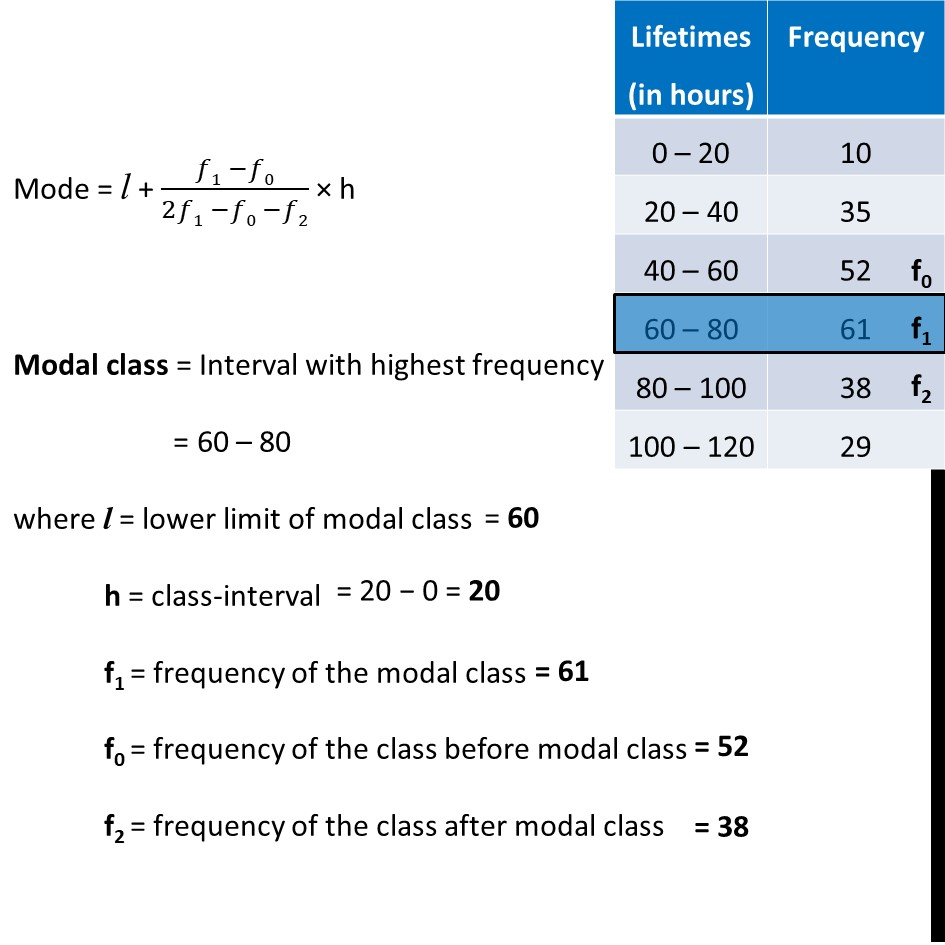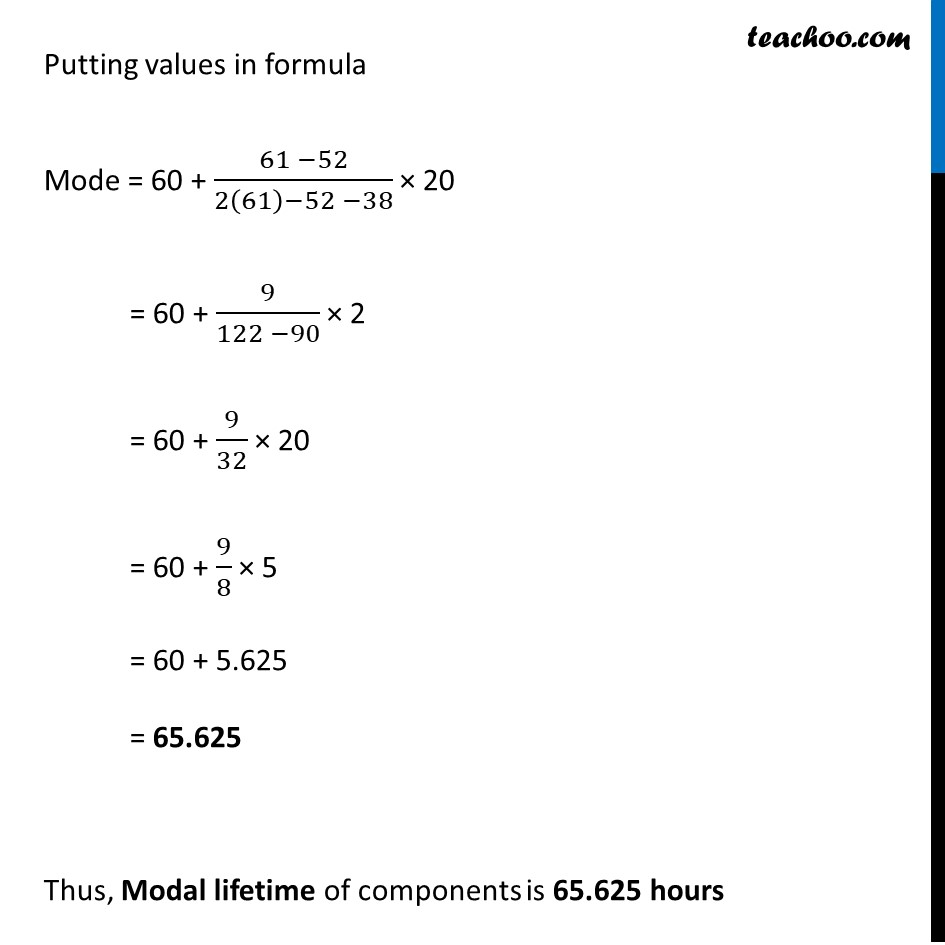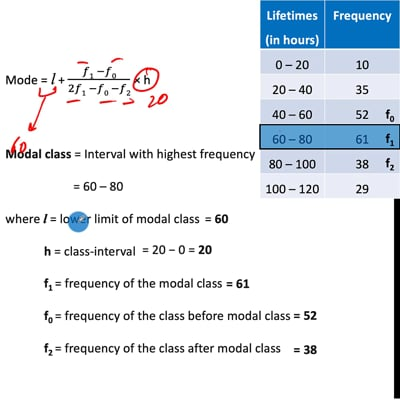Ex 14.2

Chapter 14 Class 10 Statistics
Serial order wiseThis video is only available for Teachoo black users

Solve all your doubts with Teachoo Black (new monthly pack available now!)

### Transcript

Ex 14.2, 2 The following data gives the information on the observed lifetimes (in hours) of 225 electrical components . Determine the modal lifetimes of the components. Mode = l + (𝑓1 −𝑓0)/(2𝑓1 −𝑓0 −𝑓2) × h Modal class = Interval with highest frequency = 60 – 80 where l = lower limit of modal class h = class-interval f1 = frequency of the modal class f0 = frequency of the class before modal class f2 = frequency of the class after modal class Putting values in formula Mode = 60 + (61 −52)/(2(61)−52 −38) × 20 = 60 + 9/(122 −90) × 2 = 60 + 9/32 × 20 = 60 + 9/8 × 5 = 60 + 5.625 = 65.625 Thus, Modal lifetime of components is﻿ 65.625 hours